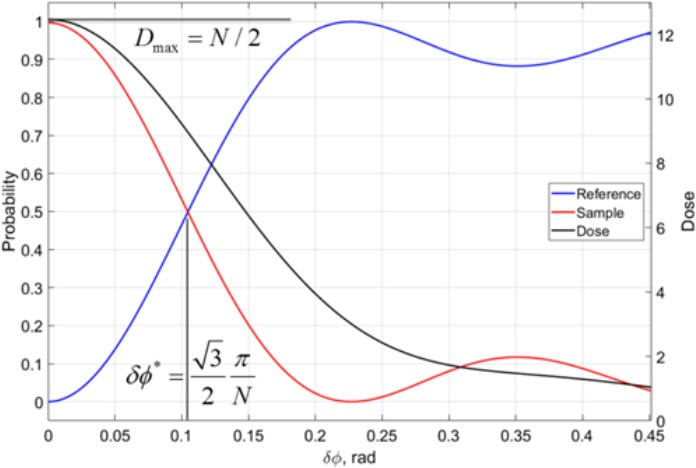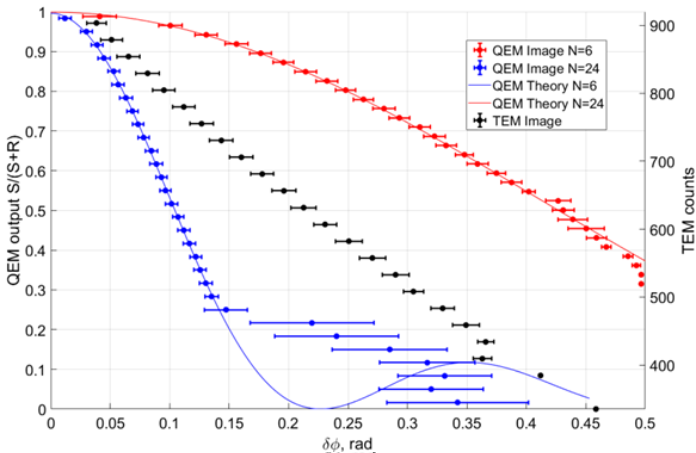# Phase Contrast in Quantum Electron Microscopy

Abstract number
520
Event
European Microscopy Congress 2020
DOI
10.22443/rms.emc2020.520
Corresponding Email
[email protected]
Session
PST.3 - New Instrumentation
Authors
Sergey V. Loginov (1), Maurice Krielaart (1), Pieter Kruit (1)
Affiliations
1. TU Delft
Keywords

Dose limited phase resolution, Interaction Free Measurements, Shot Noise

Abstract text

Quantum Electron Microscopy technique (QEM) is aimed for improvement of electron dose limited resolution by implementing interaction free measurements . One of the QEM approaches is to build a Mach-Zehnder interferometer for electrons using grated mirrors as electron beam-splitters . Earlier, it was shown that a sample with different transparency levels can be imaged by QEM in interaction free manner . Now we consider the case of phase-only sample which represents a typical cryo-EM situation. We conduct theoretical analysis on the dose limited phase resolution of QEM in order to prove it is worthwhile to build.

Cryo-Electron Microscopy suffers from sensitivity of biological samples to electron damage. Large proteins and protein complexes can be studied in cryo-EM, while for smaller proteins the X-ray diffraction is still the technique of choice. Modern aberration corrected TEMs and STEMs provide atomic resolution in crystalline samples with illumination doses higher than ~104 e-/A2. Protein structure degrades severely already at doses of two orders of magnitude lower. Novel EM techniques aim for higher dose efficiency that will allow new areas for the cryo-EM investigation. One of such techniques is QEM.

In QEM an electron is split into two states, a sample state |S> and reference state |R> so that initially <R|R>≈1. The sample state is used as a STEM focused probe while the reference state passes through the empty space. The |S>-state experiences phase shift passing through the sample  |S> →exp[i*δϕ]|S>. There is also probability for electron to collapse onto the sample in an (inelastic) interaction. The energy lost by the electron is the damage dealt to the sample. Since the electron was split so that  <S|S><<1 the probability of damage by electron is very low in the first pass. In following description inelastic interaction is omitted; experimentally such electrons can be separated from the true  |S>-state by an energy loss detector. After the sample plane, the two states are directed back to the beam-splitter (with coupling strength γ) where they exchange some of their amplitudes: |S'>=√(1-γ)exp[i*δϕ]|S> + i√ γ |R>,  |R'>=√(1-γ)|R> + i√ γ exp[i*δϕ] |S> . This cycle is repeated N(γ) times and the electron is taken out to the detector plane to collapse in the |R>-state or in the |S>-state.

The probability of collapse into the |R>-state or in the |S>-state. Then for large δϕ (see Fig.1). N(γ) can be chosen such that for δϕ=0 the electron always arrives into the |S>-state. Then for large δϕ the interference on the beam-splitter is inconsistent and probability to find the electron in the |R>-state is overwhelming. The total dose D is the |S>-state probability integrated over all N QEM cycles. The maximal dose reaches N/2 at δϕ=0 representing the fact that electron spends half of the time in the sample arm on average. At large δϕ the dose is lower since the |S>-state does not build up.Figure 1 Phase dependent behavior of a QEM with N=24 cycles. Phase shift in the sample δϕ controls collapse probabilities of electrons. For large δφ  electrons mostly locked in the initial reference state (blue). For δϕ <√3π/2N more electrons are detected in the sample sate (red). Electron dose also depends on the phase (black, right axis), with the δφ =0 receiving maximum dose of  N/2.

To compare QEM with other EM methods we estimate dose limited phase resolution. Intensity variations in an image occur either deterministically (reflecting the variations in the sample) or stochastically (shot noise). The minimal phase sensitivity Δφ should be so great that the change in intensity is comparable with the shot noise: Δφ|dC/dφ|I=√C(φ)I, where C(φ) is the contrast function and I is the average number of electrons per pixel. Hence for C(φ)I electrons detected the phase shift of the sample is within interval [φ-Δφ; φ+Δφ] with probability 63%. Note that Δφ is proportional to 1/√γ just as SNR in the shot noise limited measurements. For different microscopy techniques phase resolution can be written as Δφ =F/√γ, where F is a (technique dependent) factor and D is the illumination dose (which is not equal to I in case of QEM). For a TEM with an ideal Zernike phase plate FTEM=1/2. If we use the upper estimate for the dose in QEM Dmax=I*N/2 then FQEM≈1.6/√N. With image simulations we verify that QEM achieves the same phase resolution as TEM with an ideal Zernike phase plate at N=6 and outperforms it factor of two at N=24 (see Fig.2). In a multi-pass TEM FmTEM=1/2√N however the dose is distributed uniformly and the contrast function can become ambiguous at large N .Figure 2 Comparison of phase estimation based on noisy images with the illumination dose of 800e-/pixel. QEM at N=6 (red) gives approximately the same phase error as TEM (black, right axis) and QEM at N=24 (blue) gives error twice lower.

QEM is a STEM technique which is able to measure sample phase. QEM has the unique property: the electron dose depends on the phase value. We conclude that QEM can achieves same dose limited phase resolution as an ideal TEM with a Zernike phase plate when the number of cycles is and has a higher dose limited phase resolution at higher number of cycles .

References

 P.Kruit et al, Ultramicroscopy Volume 164 (2016), pp. 31-45

 M.A.R. Krielaart and P. Kruit, Phys. Rev. A 98 063806 (2018), pp. 1-5

 S. Thomas et al, Phys. Rev A 90, 053840 (2014), pp. 1-10

 T. Juffmann et al, Scientific Reports 7: 1699 (2017), pp 1-7

 The authors acknowledge funding from Gordon and Betty Moore Foundation